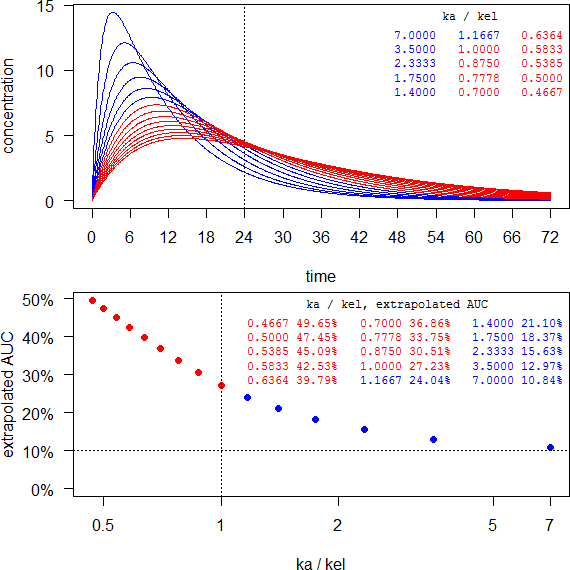## EMA: Waiving MD study [Regulatives / Guidelines]

Dear all,

at the end anscript to play with.

For any elimination half life longer than ~7 hours and an intended dosing interval of 24 hours it will be impossible to get the MD study waived. Red are formulations with flip-flop PK (kakel).

last <- 72 ke   <- log(2)/7 tlag <- 0If the half life is short (say, 3 hours) you don’t have to dive deep into flip-flop PK and may succeed. However, such a limited extension might not match your development target.

last <- 36 ke   <- log(2)/3 tlag <- 1C.t <- function(f, D, V, ka, ke, t, tlag) { # 1-compartment   if (any(c(length(f) > 1, length(D) > 1, length(V) > 1,             length(ka) > 1, length(ke) > 1)))     stop("f, D, V, ka, ke have to be scalars.")   if (f <= 0 | f > 1) stop("f has to be positive <= 1.")   if (any(c(D <= 0, V <= 0, ka <= 0, ke <= 0)))     stop("D, V, ka, ke have to be positive.")   if (missing(tlag)) tlag <- 0   if (tlag < 0) stop("tlag has to be >= 0.")   if (ka != ke) {     C <- f*D*ka/(V*(ka-ke))*(exp(-ke*(t-tlag))-exp(-ka*(t-tlag)))   } else { # flip-flop PK     k <- ka     C <- f*D*k*(t-tlag)/V*exp(-k*(t-tlag))   }   C[C < 0] <- NA # negatives due to lag-time   return(C) } AUC.t <- function(C, t, tau) { # lin-up/log-down method   t <- t[t <= tau]             # for AUC until tau   AUC.t <- 0   for (j in 2:length(t)) {     if (!is.na(C[j-1])) {       if (C[j] >= C[j-1]) { # linear-up         AUC.t <- AUC.t+0.5*(t[j]-t[j-1])*(C[j]+C[j-1])       } else {              # logarithmic-down         AUC.t <- AUC.t+(t[j]-t[j-1])*(C[j]-C[j-1])/log(C[j]/C[j-1])       }     }   }   return(AUC.t) } AUC.i <- function(AUC.tau, C, t, last, tau) { # extrapolation   tlast.3  <- tail(t[t <= last], 3)   Clast.3  <- tail(C[t <= last], 3)   Clast    <- tail(Clast.3, 1)   Ctau     <- C[t == tau]   mod      <- lm(log(Clast.3) ~ tlast.3)   lambda.z <- -coef(mod)[]   if (lambda.z <= 0) stop("increasing concentrations")   AUC.inf  <- AUC.tau+Ctau/lambda.z   return(AUC.inf) } last  <- 72 tau   <- 24 f     <- 1 D     <- 100 V     <- 5 ke    <- log(2)/7       # moderate elimination half life tlag  <- 0              # optional >= 0 forms <- 15             # no. of formulations ka    <- log(2)/1:forms # vary absorption t     <- seq(0, last, 0.1) AUC.ext <- numeric() windows(width = 6, height = 6) op    <- par(no.readonly = TRUE) par(mar = c(4, 4, 0.2, 0) + 0.1, family = "sans") split.screen(c(2, 1)) screen(1)   for (j in 1:forms) {     C <- C.t(f, D, V, ka[j], ke, t, tlag)     if (j == 1) {       plot(t, C, type = "l", col = "blue", axes = FALSE,            xlab = "time", ylab = "concentration")       abline(v = tau, lty = 3)       axis(1, labels = seq(0, last, 6), at = seq(0, last, 6))       axis(2, las = 1)     } else {       if (ka[j] > ke) {         lines(t, C, col = "blue")       } else { # flip-flop         lines(t, C, col = "red")       }     }     AUC.tau    <- AUC.t(C, t, tau)     AUC.inf    <- AUC.i(AUC.tau, C, t, last, tau)     AUC.ext[j] <- (AUC.inf-AUC.tau)/AUC.inf   }   clr <- ifelse (ka/ke > 1, clr <- "blue", clr <- "red")   par(family = "mono")   legend("topright", title = "ka / kel", box.lty = 0,          legend = sprintf("%.4f", ka/ke), title.col = "black",          text.col = clr, cex = 0.75, ncol = 3); box() screen(2)   par(family = "sans")   plot(ka/ke, 100*AUC.ext, ylim = c(0, max(100*AUC.ext)), log = "x",        axes = FALSE, xlab = "ka / kel", ylab = "extrapolated AUC",        pch = 19, col = clr)   points(rev(ka/ke)[which(rev(AUC.ext) <= 0.1)],          rev(100*AUC.ext)[rev(AUC.ext) <= 0.1], pch = 21, col = "#00C000",          bg = "transparent", cex = 1.55)   xticks <- c(axTicks(side = 1, log = TRUE), max(ka/ke))   axis(1, at = xticks, labels = xticks)   axis(2, las = 1, at = c(0, pretty(100*AUC.ext)),        labels = sprintf("%.0f%%", c(0, pretty(100*AUC.ext))))   abline(v = 1, lty = 3)  # flip-flop limit   abline(h = 10, lty = 3) # MD waiver limit   par(family = "mono")   legend("topright", title = "ka / kel, extrapolated AUC", box.lty = 0,          legend = sprintf("%.4f %.2f%%", rev(ka/ke), rev(100*AUC.ext)),          title.col = "black", text.col = rev(clr), cex = 0.75, ncol = 3,          bg = "white"); box() close.screen(all = TRUE) par(op)

Dif-tor heh smusma 🖖🏼 Довге життя Україна!Helmut SchützThe quality of responses received is directly proportional to the quality of the question asked. 🚮
Science QuotesIng. Helmut Schütz